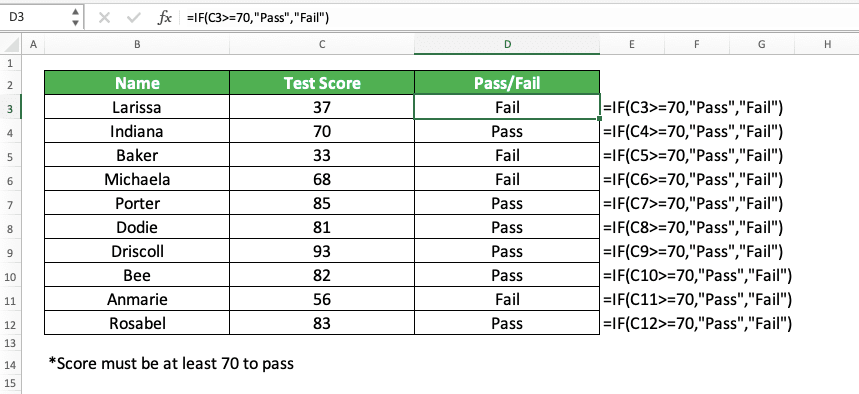How to Write an IF Formula for Pass/Fail in Excel - Compute Expert

# How to Write an IF Formula for Pass/Fail in Excel

Home >> Excel Tutorials from Compute Expert >> Excel Tips and Trick >> How to Write an IF Formula for Pass/Fail in Excel

In this tutorial, you will learn how to write an IF formula to determine the pass/fail of test scores completely.

When processing test scores in excel, we might sometimes need to determine their pass/fail marks. We usually base this on the minimum passing score we have for those test scores.

If they equal or exceed our minimum passing score, then we consider them to pass. Otherwise, if they are below our minimum passing score, then we consider them to fail. If we know how to write the correct IF formula to determine that, we can get our “pass/fail” marks easily.

Want to know the way to write the IF formula to determine the pass/fail of a test score in excel? Read this tutorial until its last part!

Disclaimer: This post may contain affiliate links from which we earn commission from qualifying purchases/actions at no additional cost for you. Learn more

## How to Write an IF Formula for Pass/Fail in Excel

The way to write an IF formula to determine pass/fail in excel is quite simple. If you understand the way to use number operators in a logic condition, you should be able to write it.

Here is the general way to write the IF formula for this pass/fail mark purpose.

= IF ( test_score >= minimum_passing_score , “Pass” , “Fail” )

In this writing, we tell our IF to compare our test score with our minimum passing requirement of the test score. If it is equal to or more than the minimum passing score, then our IF will produce “Pass”. If otherwise, our IF will produce “Fail”.

To better understand the formula writing concept, here is its implementation example in excel.As you can see there, we can get the “pass/fail” mark of our test scores from the IF formula writing.

Just compare the test score with the minimum passing score and input the “Pass” and “Fail” marks inside the IF. After we finish writing the IF for the first test score, just copy the formula to mark all the test scores. By doing that, we will immediately get all the pass/fail marks that we need.

## How to Write an IF Formula for Pass/Retest/Fail in Excel

What if we have the option to retest also for a certain test score range? If that is the case, then we just need to write two IFs and put one in another. Input the retest mark condition in one of the IFs.

Here is the general writing form of the nested IFs for this purpose in excel.

= IF ( test_score >= minimum_passing_score , “Pass” , IF ( test_score >= minimum_retest_score , “Retest” , “Fail” ) )

In this IF writing, we input the logic condition for passing in the first IF. This IF will catch all the test scores that pass. Thus, we just need to compare the test score with the minimum retest score in the second IF.

If the test score doesn’t fulfill the requirements to pass or retest, our IF will produce the “Fail” mark.

Here is the implementation example of this IF formula writing in excel.For the previous set of test scores, we have a new retest score requirement. Thus, we write nested IFs and we compare the test score with the minimum retest score in the second IF. By doing that, we can mark the test scores which need to take retest too!

## Exercise

After you have learned how to write an IF formula to determine the pass/fail in excel, let’s do an exercise. This is so you can understand the tutorial lessons more practically.

### Question

Answer all the questions below in the appropriate gray-colored cell according to the question number!
1. The passing requirement for the test score is 65. What is the pass/fail mark of each test score?
2. We add the chance to retest for the students and the retest requirement is 55. What is the pass/retest/fail mark of each test score?
3. We increase the passing and retest requirement for the test score to 75 and 60. What is the pass/retest/fail mark of each test score?

You can also use IF if you want to grade test scores into letters (A, B, C, D, E, etc). Just change the “Pass”, “Retest”, and “Fail” marks to those letters. Add more IFs into your nested IFs if you need them.

Related tutorials you should learn too:

Get updated excel info from Compute Expert by registering your email. It's free!